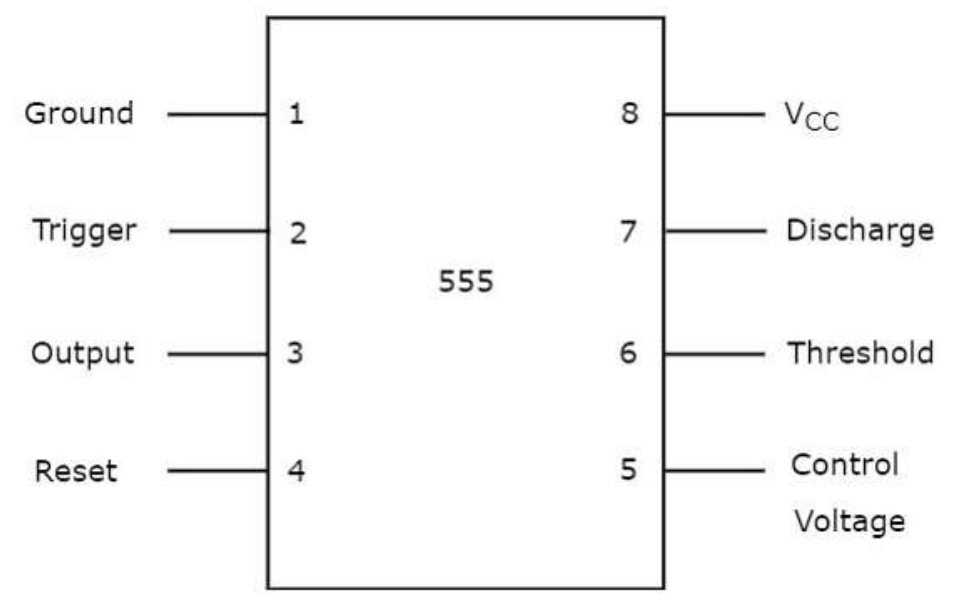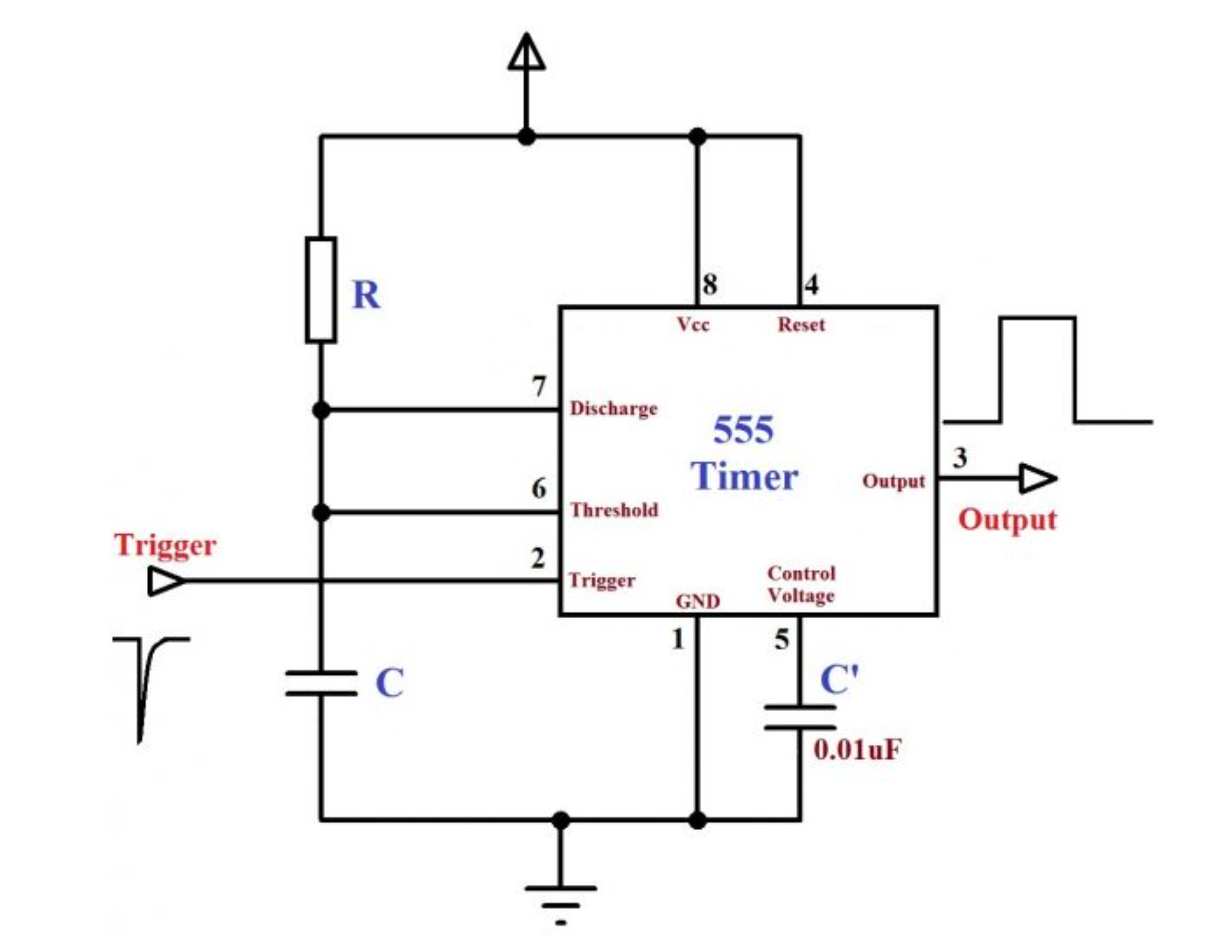Select Page

# Timer IC and Multivibrators

Analog Circuits: Timer IC and Multivibrators

Contents

Describe the internal diagram of 555 Timer IC 1

Recall the applications of 555 Timer IC 3

Describe the Pin diagram of Monostable Multivibrator using 555 Timer IC 3

Calculate the Pulse Width in Monostable Multivibrator 5

Describe the Pin Diagram of Astable Multivibrator using 555 Timer IC 6

Generate Square Wave using Astable Multivibrator 8

Calculate the Time period and Duty Cycle of Generated Square Wave 9

Generate Triangular Wave using Astable Multivibrator 10

# Describe the internal diagram of 555 Timer IC

The 555 Timer IC is a widely used integrated circuit that is commonly used in timing, oscillator, and flip-flop applications. The internal diagram of the 555 Timer IC is shown below:The 555 Timer IC contains three 5kΩ resistors (RA, RB, and RC), two comparators (COMP1 and COMP2), a flip-flop (FF), a discharge transistor (DIS), and an output stage.

The three 5kΩ resistors are used to set the voltage levels at the inputs of the comparators. The comparator inputs are labeled as TRIG (trigger) and THR (threshold). When the voltage at the TRIG input falls below two-thirds of the voltage at the THR input, the output of COMP1 goes high and triggers the flip-flop.

The flip-flop output is connected to the output stage, which can be configured in a variety of ways depending on the application. The output stage can be used as a buffer or as a switching output, and can drive loads up to 200mA.

The discharge transistor is used to discharge the capacitor connected to the DIS (discharge) pin, which is typically used to control the timing of the 555 Timer IC. The CONTROL pin can be used to modify the operation of the internal comparators and flip-flop.

The RESET pin is used to reset the internal flip-flop and return the output stage to its default state. The GND pin is connected to ground, and the Vcc pin is connected to a positive voltage source.

Overall, the 555 Timer IC is a versatile and widely used integrated circuit that offers a wide range of timing and control capabilities.

# Recall the applications of 555 Timer IC

The 555 timer IC is a versatile integrated circuit that has a wide range of applications in electronics. Some of the common applications of the 555 timer IC are:

1. Astable Multivibrator: The 555 timer IC can be used as an astable multivibrator to generate a continuous stream of pulses. This is useful in applications such as oscillator circuits, timing circuits, and LED blinkers.
2. Monostable Multivibrator: The 555 timer IC can also be used as a monostable multivibrator to generate a single pulse of a specific duration. This is useful in applications such as timers, pulse width modulation circuits, and pulse position modulation circuits.
3. Voltage Controlled Oscillator (VCO): The 555 timer IC can be used as a voltage-controlled oscillator to generate a variable-frequency output signal that is controlled by an external voltage.
4. PWM Generator: The 555 timer IC can be used as a pulse-width modulation (PWM) generator to control the speed of motors, dim the brightness of LEDs, and regulate the power output of power supplies.
5. Tone Generator: The 555 timer IC can be used as a tone generator to produce different types of audio signals such as square waves, sawtooth waves, and triangular waves.
6. Delay Circuits: The 555 timer IC can be used to generate delays in circuits such as relay timers, digital clocks, and time delay switches.
7. Flip-Flop Circuits: The 555 timer IC can be used to implement flip-flop circuits, which are widely used in digital electronics.

Overall, the 555 timer IC is a versatile component that is widely used in electronic circuits due to its simplicity, low cost, and ease of use.

# Describe the Pin diagram of Monostable Multivibrator using 555 Timer IC

A Monostable Multivibrator using a 555 Timer IC is a circuit that generates a single output pulse of a specified duration when triggered. The Pin diagram of a Monostable Multivibrator using 555 Timer IC is shown below:The Monostable Multivibrator circuit using a 555 Timer IC has the following pins:

1. GND: This is the ground pin of the 555 Timer IC.
2. Trigger (Trig): This is the input pin used to trigger the Monostable Multivibrator. When this pin is brought to a voltage level lower than 1/3 of the supply voltage, the output of the 555 Timer IC goes high.
3. Output: This is the output pin of the Monostable Multivibrator. When triggered, it goes high for a duration determined by the external RC timing components.
4. Reset: This is the input pin used to reset the Monostable Multivibrator. When this pin is brought to a voltage level lower than 0.7 volts, the output goes low and the timer is reset.
5. Control: This pin is used to control the operation of the internal comparators and flip-flops.
6. Threshold: This is the input pin used to set the voltage level at which the output of the 555 Timer IC goes high. This voltage level is set using an external voltage divider circuit.
7. Discharge: This is the output pin used to discharge the external capacitor that determines the pulse duration.

Overall, the Monostable Multivibrator using a 555 Timer IC is a simple and commonly used circuit that generates a single output pulse of a specified duration when triggered, and its pin diagram helps understand its working and operation.

# Calculate the Pulse Width in Monostable Multivibrator

The pulse width of a Monostable Multivibrator is determined by the values of an external resistor (R) and capacitor (C) connected to the 555 Timer IC. The pulse width can be calculated using the formula:

Pulse Width (t) = 1.1 x R x C

where t is the pulse width in seconds, R is the resistance in ohms, and C is the capacitance in farads.

For example, let’s say we have a Monostable Multivibrator with an external resistor of 10k ohms and a capacitor of 10 microfarads. Using the above formula, we can calculate the pulse width as:

t = 1.1 x 10k ohms x 10 microfarads

t = 110 microseconds

Therefore, the pulse width of the Monostable Multivibrator in this example is 110 microseconds.

# Describe the Pin Diagram of Astable Multivibrator using 555 Timer IC

An Astable Multivibrator using a 555 Timer IC is a circuit that generates a continuous stream of square wave pulses with a frequency determined by the values of an external resistor and capacitor. The Pin diagram of an Astable Multivibrator using 555 Timer IC is shown below: